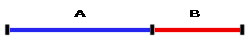# Golden Ratio Calculator

## Calculating Missing Values Using the Golden Ratio Calculator

Identifying a missing value can be complex and time-consuming. We have created the Golden Ratio Calculator to enable you to swiftly and effortlessly apply the Golden Ratio to find a missing value.

Simply enter the total length value of A and B, or alternately, enter the lengths of either A or B and click "Calculate" to find the remaining two values.

## The Golden RatioWritten as φ, the Greek letter phi, the Golden Ratio is an irrational value that can be found by cutting a line into two sections whereby the longer section A divided by the shorter section B is equivalent to A+B (the full length of the line) divided by A. As an equation, the Golden Ratio is written as:

A/B = (A+B)/A = 1.6180339887498948420 ...

Typically, the Golden Ratio is rounded to 1.618, a figure that has continually reappeared in numerical research and, as a result, has been renamed several times as the Golden Section, the Divine Proportion, the Golden Mean and so on.

Online Golden Ratio Calculator

Enter only one value in any of the fields below:

You may set the number of decimal places in the online calculator. By default there are only two decimal places.

Results
• A/B = (A+B)/A = 1.6180339887498 ...
• A =
• B =
• A + B =
A
/
B
=
A + B
/
A
/
=
/

## The Golden Ratio Formula

Our Golden Ratio Calculator uses the following formula to identify missing values:

φ = A/B = (A+B)/A

φ = (  1 +  √5 ) / 2 = 1.6180339887498948482045868 ...

You may also be interested in our Online Ratio Calculator and Aspect Ratio Calculator